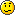Welcome to MapForums!

When you are signed in, this message, the ads in this row, and the red-underlined link ads all go away.## Importing address with an array of fields specified

This is a discussion on Importing address with an array of fields specified within the MapPoint Desktop Discussion forums, part of the Map Forums category; I am importing a list of addresses from SQL UDL. For some reason the import defaults the name of the ...

1.Junior Member White Belt
Join Date
Oct 2003
Posts
8

## Importing address with an array of fields specifiedI am importing a list of addresses from SQL UDL. For some reason the import defaults the name of the pushpin balloon to Address2 if it is not Null, otherwise it defaults to Address1. I really want it to be a user field called "Tech_ID". I tried to create an array of fields specification (see the attached code) but always get the infamous 'The Parameter is Incorrect' . I'm guessing I have something spec'd wrong on the array. Anyone give a hand on this?

Dim TechFieldArray(,) As MapPoint.GeoFieldType
TechFieldArray = New MapPoint.GeoFieldType(8, 2) {}
'TechFieldArray(1, 1) = "Tech_ID"
TechFieldArray(1, 1) = 1
TechFieldArray(1, 2) = MapPoint.GeoFieldType.geoFieldName
'TechFieldArray(2, 1) = "Current_Site"
TechFieldArray(2, 1) = 2
TechFieldArray(2, 2) = MapPoint.GeoFieldType.geoFieldData
TechFieldArray(3, 1) = 3
TechFieldArray(4, 1) = 4
'TechFieldArray(5, 1) = "City"
TechFieldArray(5, 1) = 5
TechFieldArray(5, 2) = MapPoint.GeoFieldType.geoFieldCity
'TechFieldArray(6, 1) = "State"
TechFieldArray(6, 1) = 6
TechFieldArray(6, 2) = MapPoint.GeoFieldType.geoFieldRegion1
'TechFieldArray(7, 1) = "ZIP"
TechFieldArray(7, 1) = 7
TechFieldArray(7, 2) = MapPoint.GeoFieldType.geoFieldPostal1
'TechFieldArray(8, 1) = "Country"
TechFieldArray(8, 1) = 8
TechFieldArray(8, 2) = MapPoint.GeoFieldType.geoFieldCountry

' Import the Tech Site Addresses Query view, using the TechField Array to map the fields to
' Mappoint dataset import geo field codes
oDataSetTechs = oMap.DataSets.ImportData("C:\UDL\Data_WorkbenchSQL .UDL!Tech_Site_Addresses Query", TechFieldArray)Reply With Quote

2.Junior Member White Belt
Join Date
Oct 2003
Posts
8

## Here is how it should have been codedDim JobFieldArray(,) As Object
ReDim JobFieldArray(6, 1)
JobFieldArray(0, 0) = "Job_ID"
JobFieldArray(0, 1) = MapPoint.GeoFieldType.geoFieldName
JobFieldArray(3, 0) = "City"
JobFieldArray(3, 1) = MapPoint.GeoFieldType.geoFieldCity
JobFieldArray(4, 0) = "State"
JobFieldArray(4, 1) = MapPoint.GeoFieldType.geoFieldRegion1
JobFieldArray(5, 0) = "ZIP"
JobFieldArray(5, 1) = MapPoint.GeoFieldType.geoFieldPostal1
JobFieldArray(6, 0) = "Country"
JobFieldArray(6, 1) = MapPoint.GeoFieldType.geoFieldCountry

' The next statement performs a Mappoint Import Data from the SQL table
oDataSetJobs = oMap.DataSets.ImportData("C:\JHS Varicom\DA Conversion\RDA_Data_WorkbenchSQL.UDL!Job_Cust_SLA_ Address_View", _
JobFieldArray, MapPoint.GeoCountry.geoCountryUnitedStates, MapPoint.GeoDelimiter.geoDelimiterDefault)Reply With Quote

There are currently 1 users browsing this thread. (0 members and 1 guests)

address, array, fields, importing